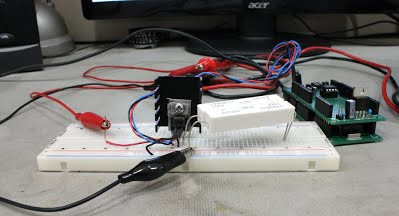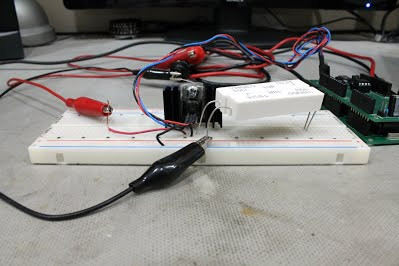Search

# UNDERSTANDING POWER AND HEATSINKS PART II - REAL WORLD EXAMPLES

Updated: Nov 17, 2020

Heat in electronics is inevitable but improper management of heat can be catastrophic. See a real world application and actual temperature changes.Last time in Part I we covered the theory behind how heat sinks remove excess heat and how to calculate the temperatures of a given part. In Part II we will examine a real world example and graph the actual temperature changes using 4 different sized heat sinks under the same load conditions. Our test circuit is simply a 12V source, 7805 +5V regulator with a TO220 case, and a 20 ohm load resistance. See the schematic below.

TEST CIRCUITTesting paramaters are as follows:

• Data will be collected over 10 minutes at 2 samples per second resulting in 1200 data points.

• Data will be measured by bonding a linear temperature sensor directly to the front of the regulator.

• Testing will be done using still air.

• Testing will start at ambient.

• 4 different heatsinks of thermal resistances of: 28, 20, 12, and 3 °C/W.

• Power dissipated is 1.75W (P = V * I = (12 - 5) * (5 / 20) = 7 * 0.25 = 1.75W)

• Regulator ratings: 150°C max junction temp, Rja 23.9°C/W, Rjc 1.7°C/W

• Themal pad with 0.35°C/W thermal resistance between regulator and heatsink.

The Four Heatsinks Used in the TestsBelow is a summary chart showing the temperature over time results for the tests conducted belowThe graph above has one additional test with no heatsink and a 40 ohm load to reference.

Test 1, No heatsink     This test is with no heatsink and the regulator in free air.     The theoretical temperature is:     T= P * Rja + Tamb = 1.75W * 23.9°C/W + 26°C = 67.8°C

Actual Values: Vin = 12.13 Vout = 4.92 I = 0.246A Power = 1.77W T = 1.77 * 23.9 + 26 = 68.4°C

From this you can see the temperature ended at 84°C after 10 minutes, well above the calculated value of 68°.

We can calculate the actual thermal resistance.     Tja = (T - Tamb) / P = (84.2 - 26) / 1.77 = 32.4°C/W     This value is 35% higher than the datasheet meaning it is less efficient!

Test 2, 28°C/W heatsink

This test is with heat sink and thermal pad.     The theoretical temperature is:     T = P * (Rjc + Thermal Pad + Heat Sink) + Tamb

= 1.75W * (1.7 + 28 + 0.35°C/W) + 26°C = 78.6°CActual Values:

Vin = 12.13

Vout = 4.87

I = 0.246A

Power = 1.79W

T = 1.79 * 30.05 + 24.4 = 78.2°C

From this you can see the temperature ended at 69°C after 10 minutes, well below the calculated value of 78°.

We can calculate the actual thermal resistance:     Tja = (T - Tamb) / P = (69.3 - 24.4) / 1.79 = 25.0°C/W     This value is 16.5% lower than the theoretical meaning it is more efficient at removing heat.

Test 3, 20°C/W heatsink

This test is with the heat sink and thermal pad.     The theoretical temperature is:     T = P * (Rjc + Thermal Pad + Heat Sink) + Tamb

= 1.75W * (1.7 + 20 + 0.35°C/W) + 26°C = 64.6°CActual Values:

Vin = 12.12

Vout = 4.92

I = 0.246A

Power = 1.77W

T = 1.77 * 22.05 + 26 = 65.0°C

From this you can see the temperature ended at 50°C after 10 minutes, well below the calculated value of 65°.

We can calculate the actual thermal resistance.     Tja = (T - Tamb) / P = (49.5 - 26.1) / 1.77 = 13.2°C/W     This value is 40% lower than the theoretical meaning it is quite a bit more efficient at removing heat!

Test 4, 12°C/W heatsink

The theoretical temperature is:     T = P * (Rjc + Thermal Pad + Heat Sink) + Tamb

= 1.75W * (1.7 + 12 + 0.35°C/W) + 26°C = 50.6°CActual Values:

Vin = 12.12

Vout = 4.92

I = 0.246A

Power = 1.77W

T = 1.79 * 14.05 + 26.6 = 51.7°C

From this you can see the temperature ended at 53°C after 10 minutes, only slightly above the calculated value of 51.7°.

We can calculate the actual thermal resistance.     Tja = (T - Tamb) / P = (53.4 - 26.6) / 1.79 = 15°C/W     This value is 6.5% above than the theoretical meaning it is only slightly less efficient and the closest yet.

Test 5, 3°C/W heatsink

This test is with no heatsink and the regulator in free air.     The theoretical temperature is:     T= P * (Rjc + Thermal Pad + Heat Sink) + Tamb = 1.75W * (1.7 + 3 + 0.35°C/W) + 26°C = 34.8°CActual Values:

Vin = 12.12

Vout = 4.92

I = 0.246A

Power = 1.77W

T = 1.77 * 5.05 + 26 = 34.9°C

From this you can see the temperature ended at 36°C after 10 minutes, slightly above the calculated value of 34.9°.

We can calculate the actual thermal resistance.     Tja = (T - Tamb) / P = (36.3 - 25.3) / 1.77 = 6.2°C/W     This value is 23% higher than the theoretical meaning it is less efficient at removing heat.

Conclusion There is quite a bit of variability between the theoretical and measured thermal resistance, both above and below, and care must be made when deciding what size of heatsink is required. Experimentation may be required to determine the best size heatsink to use for your application.